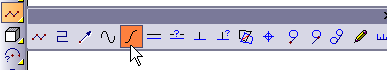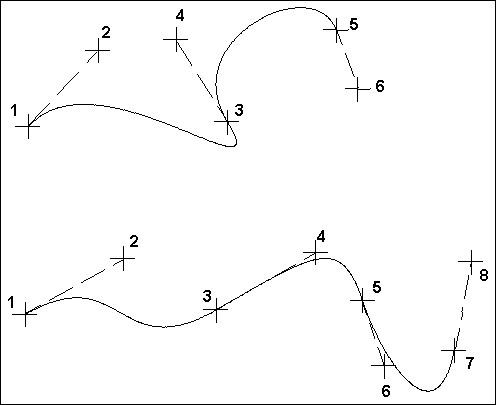# Bezier Curve

Menu: Draw / Lines / Bezier Curve

Toolbox Icon:Point 1: Beginning of the Bezier curve

Point 2: First control point for the curve(beginning tangent)

Point 3: Pass-through point

Point 4: Second control point (tangent for point 3)

Point 5: Pass-through point (optional)

Point 6: Third control point (tangent for point 5)

Point 7-n: (Optional points in pairs)

The first point is the endpoint of the curve, and the second point is the control point. The curve at the first point will be tangent to the second point. The third point is the endpoint, and the fourth point is the tangent. Each subsequent pair of points is a point for the curve to pass through and a control point to define the tangent at that location.

Moving a control point further from the curve makes the curve sharper. Points must be set in pairs. If you set an odd number of points, the last point is ignored.